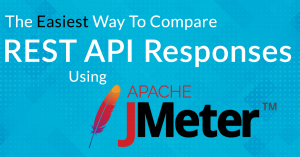Restful APIs have increased in popularity over the last few years. The trend started with giants like eBay and Amazon. This has happened because these types of APIs present multiple advantages. Some of these benefits are: good performance in component interactions, scalability, simplicity, portability and reliability.

For these types of APIs, JSON files are the default choice for formatting transferred data. These types of files are easy to read and write and can be used with any popular programming language.

As testers, API testing is something pretty common in today’s industry. These tests are performed in the message layer, since APIs lack a GUI. In a typical scenario, your team decides whether to integrate an API or design one. Before the integration with the UI is complete, the API needs to be tested. Thus, you get access to endpoints that you try various scenarios on: sending a set of parameters and asserting that the response contains the expected data. This is the most basic scenario for an API test.

Besides the case presented above, API tests can have various requirements, depending on the nature of the project and task.

One requirement I have come across recently, sounded something like: ‘We have 2 (or more) APIs. We need to check if the keys for an endpoint on API1 are exactly the same as the keys from its equivalent endpoint from API2. ‘The same’ means that the name, order and number of occurrences of each key must coincide.” The order of the of the keys in a JSON would normally not be relevant, but we will see why I mentioned it in this context.

That’s straightforward enough…

The solutions on how to test this are numerous:

The most basic solution would be to use an online JSON comparer (like this one or this one). This would imply though that you have to manually input the responses of the endpoints under test in the online comparer and repeat for every endpoint. Not too elegant if you ask me. Also, inefficient if you have to do it for hundreds of endpoints.

Another approach is to use various Java libraries that can handle these types of tasks, like Jackson or GSON.

If you choose to use Jackson, the code would go something like:

```final JSONObject obj1 = /*json*/;
final JSONObject obj2 = /*json*/;
final ObjectMapper mapper = new ObjectMapper();
return tree1.equals(tree2);```

This approach asserts if 2 JSON responses are the same. Then you can elaborate on this starting point to check more complex scenarios.

Using GSON you would need to do something like:

```JsonParser parser = new JsonParser();
JsonElement o1 = parser.parse("{a : {a : 2}, b : 2}");
JsonElement o2 = parser.parse("{b : 2, a : {a : 2}}");
assertEquals(o1, o2);```

Another viable solution is to use the JsonSlurper from Groovy which parses text into a data structure of lists and maps.

And, of course, there is the Apache JMeter™ solution.

# Comparing Rest APIs with JMeter

The JMeter approach: for the situation I presented, the API calls were already defined in the JMeter application, so it was more convenient to integrate a solution into the existing tests. The idea was to build on the existing foundation, using elements that are familiar to most JMeter users.

The first idea which comes to mind is to use the JSON Path Extractor post-processor. When it comes to extracting values from a JSON file, the JSON Path Extractor would be the optimal solution. In this situation though, for the scenario I presented where we only need to compare the keys, this type of post-processor is not a viable solution. This is because we don’t have a JSON schema for the endpoints. Also, we don’t know exactly which keys each API call contains and keys are part of the JSON Path we need to formulate. This is why, for this specific situation, we are going to use a slower and less optimal solution: the Regular Expression Extractor.

For this, minimum knowledge on regular expressions and a bit of Groovy code is required.

First, let’s try to visualise the structure of the JMeter script. Inside a thread group, there are multiple Simple Controllers, each corresponding to a group of similar endpoints that we need to compare the keys for. Inside each Controller, there are multiple (2 or more) calls to the exposed ‘sibling’ endpoints.

So, for a situation where we have 4 groups of similar endpoints each with 3 endpoints we want to compare, the JMeter tree would look something like this: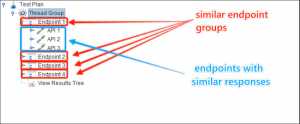Since we want to compare if all keys from the API1 JSON response are found in the responses of API2 and API3 calls, the first step is to extract them. This is done using a Regular Expression Extractor.

The expression would be “(.+?)”:. This could be translated as: match any character (the dot) except for line terminators, between one and unlimited times between the characters “ and “: literally (case sensitive).

In theory, this would extract all key names since in a JSON structure, any key is preceded by a “ character and followed by the “: characters. Then comes the corresponding value which can be (but not necessarily if the value is for example, numeric or boolean) encapsulated by doubles quotes (e.g1: “key1”:”value1”, e.g2: “key2”:value2).

The ‘Match No.’ field from the Regular Expression Extractor is set to ‘-1’ this means that JMeter will create a list with all matching results extracted by the Regex. So, if we set the ‘Name of created variable’ to ‘jsonKey’ and we have 2 results, JMeter will create the variables jsonKey_1 and jsonKey_2. The ‘Regular Expression Extractor’ will have the following format: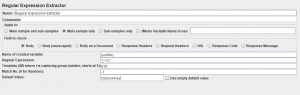The next step is to store all the results to a list. This requires 5 simple lines of code placed inside a Groovy post processor as follows:

```props.put("myList", new ArrayList())
int totalNumberOfKeys = vars.get("jsonKey_matchNr").toInteger()
for(int i=1; i<= totalNumberOfKeys; i++){
def currentKey = vars.get("jsonKey_"+i)

}```

Note: both the Regular Expression Extractor and the Groovy PostProcessor elements are children to an endpoint request, like this: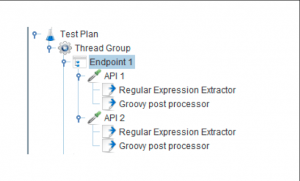Thus, for 2 endpoints with similar responses API1 and API2, after applying the elements above we would have 2 lists of keys: myList1 and myList2, each with jsonKey_1, json_key 2, etc.

The debate whether the order of the keys should be taken into account or not comes into play here. Using this regex approach, if we compare two JSON responses with different structures (which contain arrays), and the order of the keys is not calculated, the two JSONs that are different will be seen as ‘equal’. The reason is because this approach doesn’t extract the array as an object, it just fetches the keys and puts them in a list.

The last step is to compare the lists. Here we can decide if the order of the elements from the JSONs matters or not. This is simply done in a Groovy sampler using the following code which checks if the lists have the exact same keys:

```if(props.get("myList1").equals(props.get("myList2"))){
log.info("The JSON responses have the same keys and are placed in the same order")
}```

The sampler should be the last element in an endpoint group. This is because the code needs to be executed only after we have created the lists that we want to compare.

If we want to go a step further and see which keys differ, we can print them in the console (or if you want you can write the logs to a file) using the following code:

```else{
if((props.get("myList1").minus(props.get("myList2"))).size()!=0){
log.info("The JSON reponses are not equal and the mismatched keys are
(present in JSON response 1 and not JSON response 2):
"+ props.get("myList1").minus(props.get("myList2")))
}
if((props.get("myList2").minus(props.get("myList1"))).size()!=0){
log.info("The JSON reponses are not equal and the mismatched keys are
(present in JSON response 2 and not JSON response 1):
"+ props.get("myList2").minus(props.get("myList1")))
}
if((props.get("myList2").minus(props.get("myList1"))).size()==0 &&
(props.get("myList1").minus(props.get("myList2"))).size()==0){
log.info("The JSON responses have the same keys but in a different order")
}
}```

The first 2 if conditions check if there are any extra elements in either of the lists. The last “else” statement prints out a message that the key order is scrambled even if the keys are the same in all JSON responses.

To illustrate an example we will use a basic test where we compare 2 JSON responses. They are basically identical with the difference that JSON Response 1 has an additional key (‘someRandomKey’) while JSON Response 2 has another key which is not found in the first response (‘otherID’). The following screenshot draws a clear picture of what the logs would look like for the code used above, for the example we presented: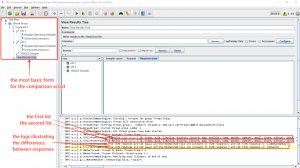For the model presented above, we would have to follow the logs to see if our test has passed or failed, since the JSR223 Sampler response will always be ‘Successful’. Technically speaking, a test has to either pass or fail. We can manipulate the Sampler to appear as ‘Failed’ if any of our conditions are not met. This is simply done by adding the following line of code into our script at the appropriate place:

`SampleResult.setSuccessful(false)`

So, for example, if we want the test to be ‘Successful’ only if the keys are the same, we would have to add ‘SampleResult.setSuccessful(false)’ in the ‘else’ statement. If the order of the keys is not important, we would add another ‘SampleResult.setSuccessful(true)’ line of code, nested in the last ‘if’ statement of the JSR223 Sampler.

If you want to use the script from the demo on your project you would just have to replace the Dummy Samplers with actual requests to various endpoints which you want to compare.

We can do this comparison for as many API calls as we want. To avoid having to write too many if statements, for that situation, you can transform the “if-else” statements above into something more general.

If we want to check all the key:value pairs to be equal in all responses we could do that as well, we would just need to change the Regex expression to “(.+?)”: (.*). But if this is the case, I would recommend using one of the approaches presented at the beginning of the article since the one with JMeter isn’t very elegant.

The conclusion is that there are always multiple approaches to a task. Sometimes you take the hard road with the purpose of learning something. Other times you use the tools in your arsenal which help you attain your goal as soon as possible and move on to the next challenge. It’s all about perspective.

For any type of inquiry or clarification, contact us at testing@evozon.com so you can clarify things with our performance engineer.